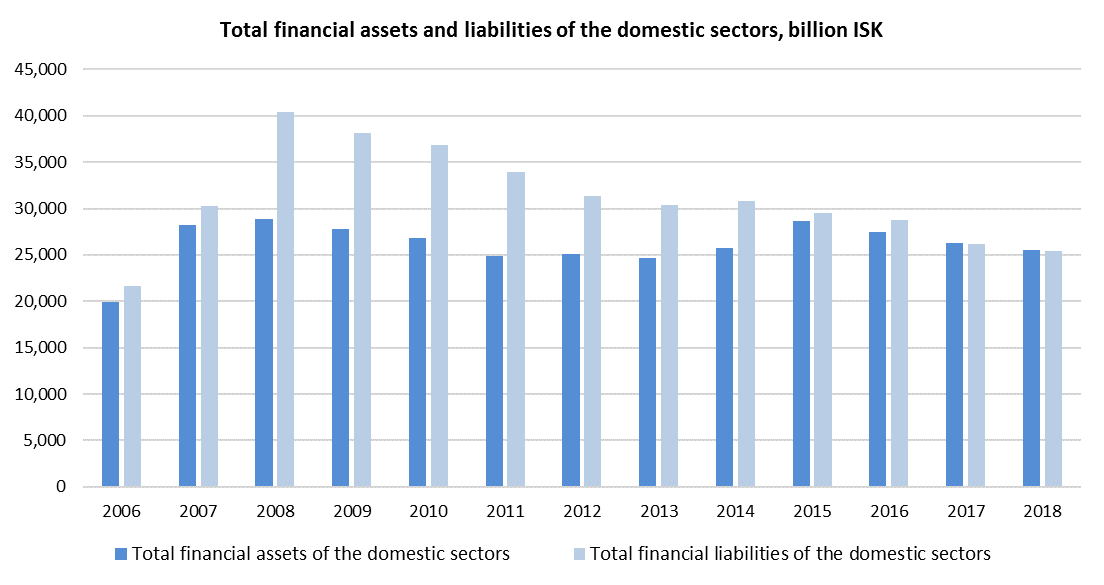Total financial assets of Iceland’s domestic sector stood at 25,519 billion ISK or 915% of Gross Domestic Product (GDP) at the end of the year 2018. Total financial liabilities stood at 25,413 billion ISK or 912% of GDP according to newly updated figures from Statistics Iceland.Financial assets of households stood at 7,212 billion ISK, while financial liabilities stood at 2,167 billion ISK at the end of 2018, or 258% and 78% of GDP, respectively.

Financial assets of non-financial corporations stood at 3,393 billion ISK according to the revised data, while liabilities stood at 7,032 billion ISK.

Total financial assets of financial corporations stood at 13,234 billion ISK, while liabilities stood at 14,214 billion ISK at the end of 2018.

At the end of 2018, the financial assets of the general government stood at 1,586 billion ISK or 57% of GDP, while liabilities stood at 1,989 billion ISK or 71% of GDP.

The foreign sector’s financial assets stood at 3,596 billion ISK or 129% of GDP, while the liabilities stood at 3,693 billion ISK or 132% of GDP.

The next publication of financial accounts will be in September 2020.

Statistics